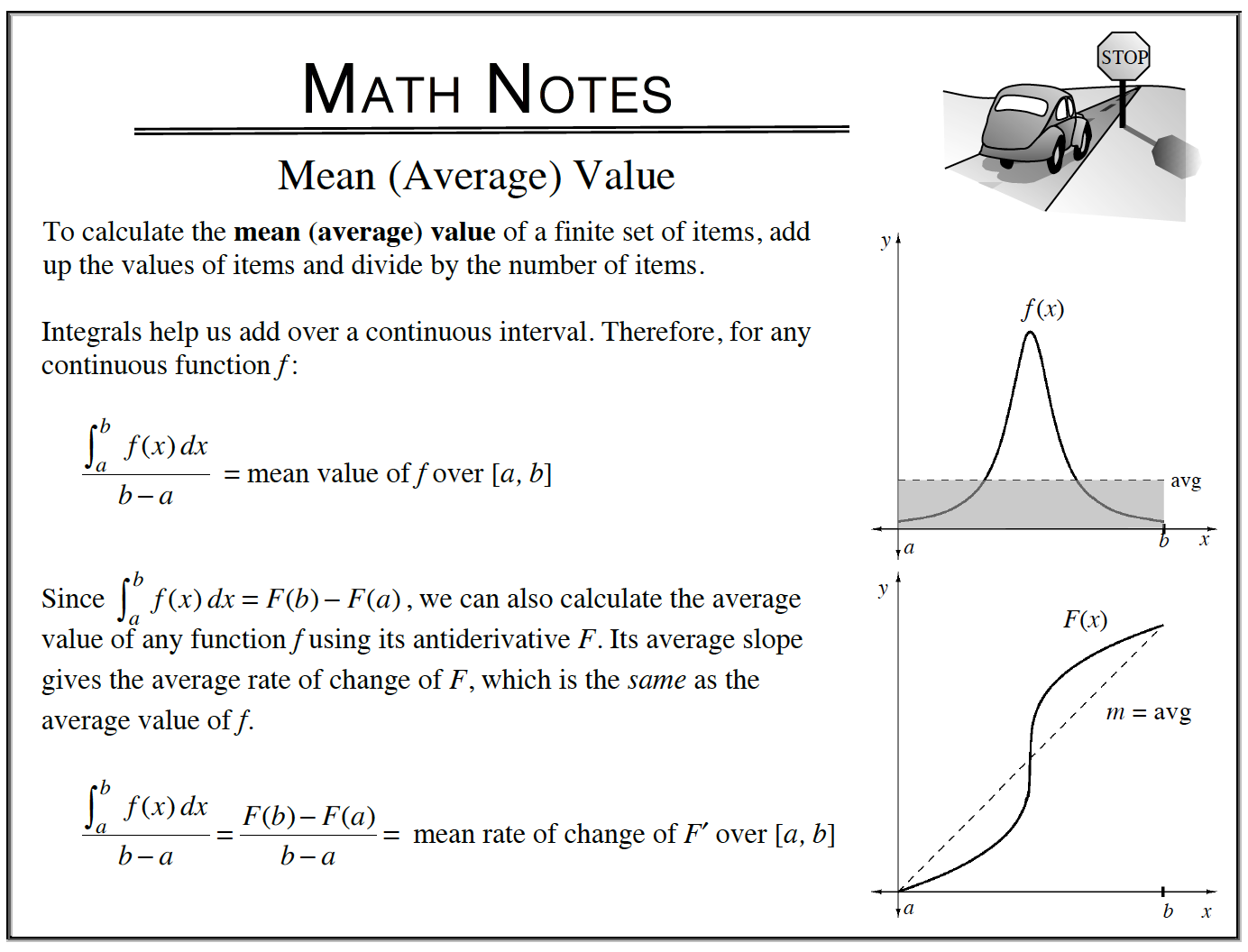### Home > CALC3RD > Chapter Ch7 > Lesson 7.2.1 > Problem7-60

7-60.

The velocity of a plane flying from San Francisco is given by $v(t) = 270\sqrt { t }$ where $t$ is measured in hours and $v(t)$ in miles per hour. Calculate the average velocity of the plane between the first and fourth hours.

You are given a velocity function and you want to find average velocity.
Should you use 'average area under the curve' or 'slope of the secant'?